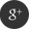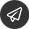| EN

## ventilation systems

The procedure below can be used to design ventilation systems:

Calculate heat or cooling load, including sensible and latent heat
Calculate necessary air shifts according the number of occupants and their activity or any other special process in the rooms
Calculate air supply temperature
Calculate circulated mass of air
Calculate temperature loss in ducts
Calculate the outputs of components - heaters, coolers, washers, humidifiers
Calculate boiler or heater size
Design and calculate the duct system
1. Calculate Heat and Cooling Loads
Calculate heat and cooling loads by

Calculating indoor heat or cooling loads
Calculating surrounding heat or cooling loads
2. Calculate Air Shifts according the Occupants or any Processes
Calculate the pollution created by persons and their activity and processes.

3. Calculate Air Supply Temperature
Calculate air supply temperature. Common guidelines:

For heating, 38 - 50oC (100 - 120oF) may be suitable
For cooling where the inlets are near occupied zones , 6 - 8oC (10 - 15oF) below room temperature may be suitable
For cooling where high velocity diffusing jets are used, 17oC (30oF) below room temperature may be suitable
4. Calculate Air Quantity

### Gallery

••••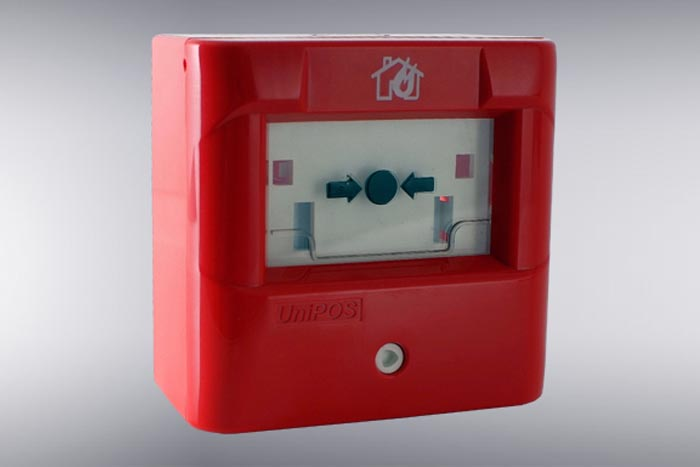•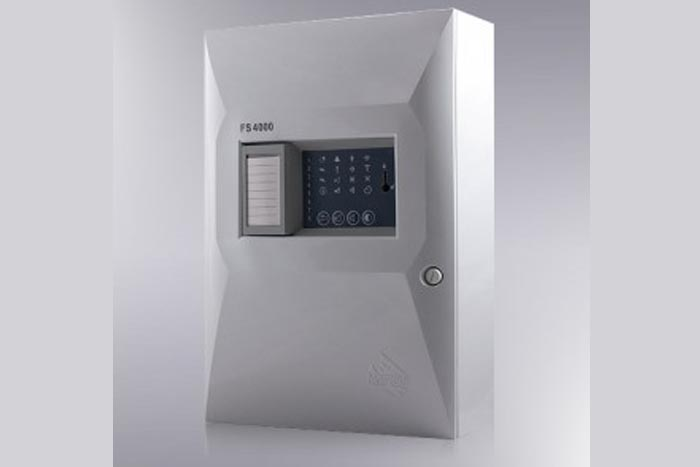•### تماس با ما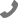021-44333678-44333679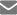hirkazhe@gmail.comتهران - شهران شمالی- بالاتر از فلکه دوم - خیابان قشلاقی-پلاک12- طبقه 4- واحد16